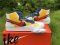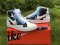USD
My Cart (0)
Home>Nike>Nike Shoes>Nike x sacai Blazer blazers

# Nike x sacai Blazer blazers Item NO: 1440604

US\$ 179.99
Colorsize
US7=UK6 1/2=FR40=JP250=CHN245 US7 1/2=UK7=FR40 2/3=JP255=CHN250 US8=UK7 1/2=FR41 1/3=JP260=CHN255 US8 1/2=UK8=FR42=JP265=CHN260 US9=UK8 1/2=FR42 2/3=JP270=CHN265 US9 1/2=UK9=FR43 1/3=JP275=CHN265 US10=UK9 1/2=FR44=JP280=CHN270 US10 1/2=UK10=FR44 2/3=JP285=CHN275 US11=UK10 1/2=FR45 1/3=JP290=CHN280 US12=UK11 1/2=FR46 2/3=JP300=CHN290
 Customized Requirements
Quantity
Stock ( 1980 )